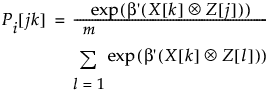Publication date: 04/12/2021

## Utility and Probabilities

Parameter estimates from the choice model identify consumer utility, or marginal utilities in the case of a linear utility function. Utility is the level of satisfaction consumers receive from products with specific attributes and is determined from the parameter estimates in the model.

The choice statistical model is expressed as follows:

Let X[k] represent a subject attribute design row, with intercept

Let Z[j] represent a choice attribute design row, without intercept

Then, the probability of a given choice for the k'th subject to the j'th choice of m choices is:where:

is the Kronecker rowwise product

the numerator calculates for the j'th alternative actually chosen

the denominator sums over the m choices presented to the subject for that trial

Want more information? Have questions? Get answers in the JMP User Community (community.jmp.com).